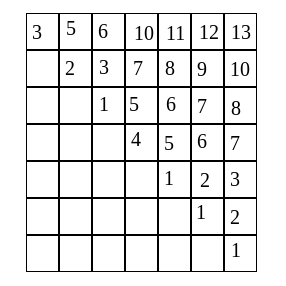For a given string of digits, find the length of the longest substring, such that the length of the substring is 2k digits, and the sum of left k digits is equal to the sum of right k digits.

Input:

The first line of input contains an integer T denoting the number of test cases. The description of T test cases follows.

Each test case contains a string of length N.

Output:

Print length of the longest substring of length 2k such that the sum of left k elements is equal to right k elements and if there is no such substring print 0.

This problem has been previously asked in Microsoft.

There are basically two approaches to solve this problem -

Approach 1) In this approach we take a window and slide it over the given string. A for loop iterates by taking different sides of the window. A second loop nested inside the first loop slides over the given string till the end of the string and a third loop is used to calculate the sum of the half left and half right elements of the string. The window size for which the sum of the left half elements and right half elements is equal is given as output. The time complexity for this approach is O(n³). But this too much. Let’s try finding a better and efficient solution.

Approach 2) In this approach we take a 2-D array and use it to store the sum of the substrings. For example, for a substring starting at i and ending at j, our 2-D matrix will contain the sum from i to j at index (i,j), i.e. sum[i][j] will give the sum of the elements of the substring from position i to j. The time complexity of this approach is O(n²).

For example — String: 3214111, the matrix formed is -The algorithm for this approach is as follows -

1. Initialize the array with the sum of substrings of length 1 at position (i,i).
2. For substrings of length ‘a’ to n repeat the steps Step-2 to Step-4.
3. Iterate diagonally in the array for the substring of length ‘a’.
4. Calculate the sum of the left half and right half of the current substring and check if they are equal. If so, then check of the length of the substring is even and if this also true update max if the current substring length > max.
5. After all the iterations return max.

Below given code is in C programming language.

```#include<stdio.h>
#include<string.h>

void longestSubstring(char *arr, int n){
int a=0, b, sum, i,j, max=0, mid;
for(a = 0 ; a < n ; a++){
sum[a][a] = arr[a] - '0';
}
for(a = 2 ; a <= n ; a++){
for(b = 0 ; b < n - a + 1 ; b++){
i = b;
j = b + a - 1;
sum[i][j] = sum[i][j-1] + sum[j][j];
mid = (i+j)/2;
if(a % 2 == 0 && sum[i][mid] == sum[mid][j] && max < a){
max = a;
}
}
}
printf("%d\n", max);
}

void main(){
char arr;
int a,n;
scanf("%s", arr);
n = strlen(arr);
longestSubstring(arr, n);
}
```